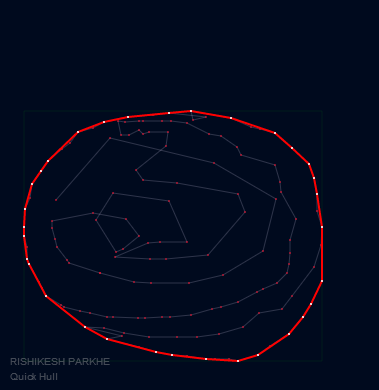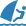Goal: To implement the fast 2D Quickhull algorithm.Another experiment on Javascript and html5 rendering. This is a well known Quickhull algorithm - which will find out an optimal bounding volume. Bounding volume is a closed volume which completely contains the points. There can be many bounding volume solutions but, we are optimising based on volume size as the criterion. This solution gives the optimal i.e. a tightest possible fitting volume.

Let us first get an overview of how this works -

1. Find the bounding box
2. Find the points lying on the box, they form a quad..
3. Eliminate the points lying inside the formed boundary
4. Find the farthest point away from each edges and form a triangle of that edge with the point.
5. Eliminate the points lying inside the triangle and add the two new edges to the hull
6. Repeat the steps 3-5 till no points are found outside the hull.Adventures in javascript

Finally, got the hang of OOP in javascript! It is quite simple to create classes (objects only) in javascript. One has to use the Java Script Object Notation (JSON).

``````//******************************************
// Vector class
//******************************************
function CVector(x, y) {
this.x = x;
this.y = y;

//-----------------------------------
// The Constructor
//-----------------------------------
this.Create = function (ptA, ptB) {
this.x = ptB.x - ptA.x;
this.y = ptB.y - ptA.y;

return this;
}
}``````

The public functions for the classes are created by the prototype methods.

``````// Dot product of two vectors
CVector.prototype.Dot = function (other) {
var product = this.x * other.x + this.y * other.y;
return product;
}

// Compute magnitude of this vector
CVector.prototype.Mag = function () {
return Math.sqrt(this.x * this.x + this.y * this.y);
}``````

The quickhull works! I had to write quite a bit of code for even this simple algorithm. Classes like CVector, CPoint, CEdge and CHull are written. Perhaps, a reusable good js geometry util can be written for general usage :-)

Don’t use the code for some serious stuff.. its come out of casual coding and might have some errors. Some escape sequences and optimizations remain, but as for the javascript experiment I am happy with it…

Have a go at it below. Enjoy :-) [works on Google Chrome]